# GSEB Solutions Class 11 Chemistry Chapter 8 Redox Reactions

Gujarat Board GSEB Textbook Solutions Class 11 Chemistry Chapter 8 Redox Reactions Textbook Questions and Answers.

## Gujarat Board Textbook Solutions Class 11 Chemistry Chapter 8 Redox Reactions

### GSEB Class 11 Chemistry Redox Reactions Text Book Questions and AnswersQuestion 1.
Assign oxidation number to the underlined elements in each of the following species.
(a) NaH2PO4
(b) NaHSO4
(c) H4P2O7
(d) K2MnO4
(e) CaO2
(f) NaBH4
(g) H2S2O7
(h) KAl(SO4)2 . 12H2O
(a) Let the oxidation number of P be x. Writing the oxidation number of each atom above its symbol we have,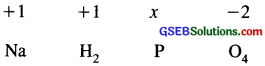sum of oxidation number of various atom in NaH2PO4
= 1(+1) 4- 2(+1) + 1 (x) + 4 (-2) = 0
But the sum of oxidation number of various atoms in NaH2PO4 is zero.
x – 5 = 0
or x = + 5
oxidation number of P in NaH2PO4 = +5

(b) Let the oxidation number of S be x.
+ 1 + 1 + x + 4 (- 2)
= 0 + 2 + x – 8 = 0
x = +6
oxidation number of S in NaHSO4 is +6.

(c) Let the oxidation number of P be x.
4(+1) + 2x + 7 (- 2) = 0
+ 4 + 2x – 14 = 0
2x = 10
x = +5
oxidation number of P in H4P2O7 = + 5.

(d) Let the oxidation number of Mn be x.
2(+1) + x + 4 (- 2) = 0
+ 2 + x – 8 = 0
x = +6
oxidation number of Mn in K2MnO4 = +6.

(e) Let the oxidation number of oxygen in CaO2 be x.
+2 + 2x = 0
2x = – 2
x = – 1
oxidation number of O in CaO2 = -1

(f) Let the oxidation number of B in NaBH4 be x.
+ 1 + x + 4(-1) – 0
x – 3 = 0
x = +3
oxidation number of B in NaBH4 = +3

(g) Let the oxidation number of S in H2S2O7 be x.
2(+1) + 2x + 7(-2) = 0
+2 + 2x – 14 = 0
2x = 12
x = +6
oxidation number of S in H2S2O7 = + 6Question 2.
What are the oxidation number of the underlined elements in each of the followings & how do you rationalise your result?
(a) KI3
(b) H2S4O6
(c) Fe3O4
(d) CH3CH2OH
(e) CH3COOH
(a) Oxidation of I in KI3 is
KI3 is made up of K+I + I2
oxidation number of I in KI is -1 whereas it is O in I2
The oxidation number of I in KI3 is = – 1

(b) Oxidation number of S in H2 S4O6 is
structure of H2 S4O6 is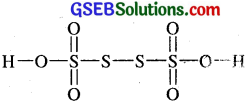The oxidation number of each of S atom linked with each other in the middle is zero while that of each of me remaining two S atom is +5.

(c) Oxidation number of Fe in Fe3O4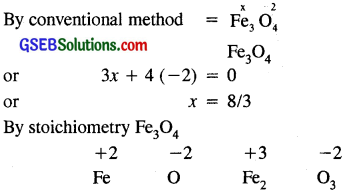Fe has oxidation number of +2 and +3.

(d) CH3CH2OH
oxidation number of carbon atom can be calculated as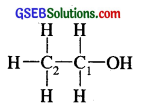C2 is attached to three H-atom (less electronegative than – c) and one – CH2OH (more electronegative than C) oxidation number of C2 is 3(+1) +x + 1 (-1) = 0
+ 3 + x – 1 = 0
x = – 2
[For each covalent bond between two dissimilar atom, assign an oxidation number of +1 to the less electronegative atom and – 1 to the more electronegative elements]
C1 is attached to one OH (O. No. = – 1) one – CH3 group (O. No. + 1), two H atom (O. No. = +1) O. No. of C1 is
+ 1 + 2(+1) + x – 1 = 0
+ 1 + 2 + x – 1 = 0
x = – 2

(e) Let us calculate the oxidation no of two atom C1 & C2 in acetic acid.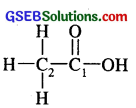C2 is attached to three H atom and one COOH group.
oxidation number of C2 is 3(+1) +x + 1 (- 1) = 0
or x = – 2
oxidation dumber of C2 = – 2
C1 is however attached to one O-atom by a double bond,
one OH group, (oxidation number = – 1) and one CH3
group (oxidation no. = +1)
oxidation number of C1 is +1 + x + (- 2) +1( – 1) = 0
x = +2
oxidation number of C1 is = +2

Question 3.
Justify that the following reactions are redox reactions :
(a) CuO(S) + H2(g) → Cu(S) + H2O(g)
CuO has been reduced to Cu whereas H2 has been oxidised to H2O.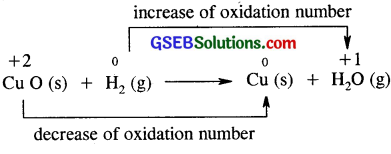(b) Fe2O3(S) + 3CO(g) → 2Fe(S) + 3CO2(g)
Fe2O3 has been reduced to Fe whereas CO has been oxidised to CO2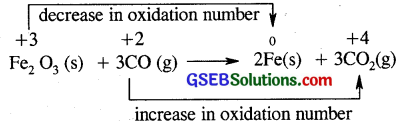Fe2O3 has been reduced to Fe, because there has occured a decrease in its oxidation number whereas CO has been oxidised to CO2, because there has occured as increase in its oxidation number.

(c) 4BCl3(g) + 3LiAlH4(S) → 2B2H6 (g) + 3LiCl(s) + AlCl3
Here BCl3 is reduced to B2H6 whereas both Li and Al have both been oxidised (addition of electronegative element chlorine).

(d) 2K(S) + F2(g) → 2K+F(S)
Here K is oxidised to K+F (addition electronegative element F) where F2 is reduced to K+F faddition of electro positive element K).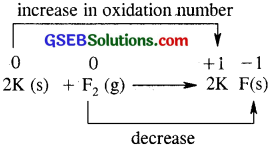K has been oxidised (increase in oxidation number). F2 has been reduced (decrease in oxidation number).

(e) 4NH3(g) + 5O2(g) → 4NO(g) + 6H2O(g)
Here NH3 has been oxidised to NO. (removal to Hydrogen, addition of oxygen) where as O2 has been reduced to H2O (addition of hydrogen).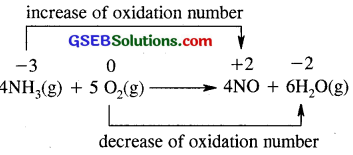NH3 has been oxidised to NO (oxidation number of N has increased from – 3 to + 2) whereas O2 has been reduced to H2O (decrease of oxidation number).

Question 4.
Fluorine reacts with ice and result in the change.
H2O(s) + F2(g) → HF(g) + HOF(g)
Justify this reaction is a redox reaction.
F undergoes reduction to form HF. Whereas F undergoes oxidation to HOF. F2 undergoes oxidation as well as reduction. It is a disproportionation reaction.
HOF is an unstable compound and decompose on its own to form H2O and OF2
2HOF → H2O + OF2.
Fluorine as such does not undergo disproportionation as it can show only one stable oxidation state of -1.
(F2 can undergo only reduction and not oxidation).Question 5.
Calculate the oxidation number of sulphur, chromium and nitrogen in H2SO5, Cr2O2-7 and NO3 . Suggest structure of these compounds. Count for the fallacy.
Oxidation number of s in H2SO5 can be calculated by understanding its structure.
(i) By conventional method.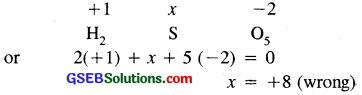But this is wrong as the maximum oxidation number of sulphur cannot be more than +6, since, it has only six valence electrons. The structure of H2SO5 is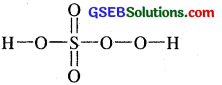in which two oxygen atoms are joined by peroxide linkage.
The oxidation number of s can be calculated as
2( +1) + x + 3(- 2) + 2(-1) = 0
for H (for O – O)
+ 2 + x – 6 – 2 = 0
x = +6
oxidation number of s in H2SO5 = + 6

(ii) Oxidation number of Cr in CrO5 By conventional method
Crx O-25
or x + 5 (- 2) = 0
x = +10
This is not possible as Cr has five 3d electrons and one 4s electrons.
Max oxidation number of Cr cannot be more than six.
In CrO5 out of 5 oxygen atoms, 4 oxygen atoms are linked as two peroxide bonds as shown.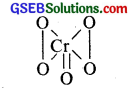oxidation number of Cr is x + 4(- 1) + 1(- 2) = 0
x – 4 – 2 – 0
x = +6
oxidation number of Cr in CrO5 is +6.

(iii) Oxidation number of N in NO3
Let oxidation number of N in NO3 = x
or x + 3(- 2) = – 1
x = +5
∴ oxidation number of N in NO3 = +5
Structure of NO3 ions is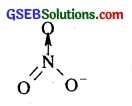Question 6.
Write formulas for the following compounds :
(a) Mercury (II) chloride : Hg (II) Cl2
(b) Nickel (IV) sulphate : Ni (II) SO4
(c) Tin (IV) oxide : Sn(IV) O2
(d) Thallium (I) Sulphate : Tl2(l) SO4
(e) Iron (III) Sulphate : Fe2(III) [SO4]3
(f) Chromium (III) Oxide : Cr2(III) O3

Question 7.
Suggest a list of the substance where. Carbon can exhibit oxidation state from – 4 to +4 and nitrogen from – 3 to +5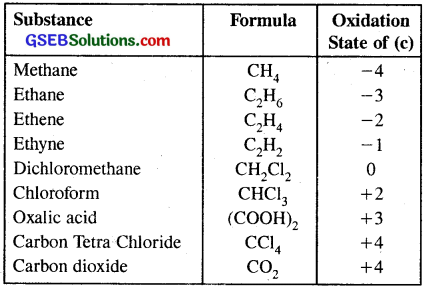substance containing N showing oxidation states from -3 to +5.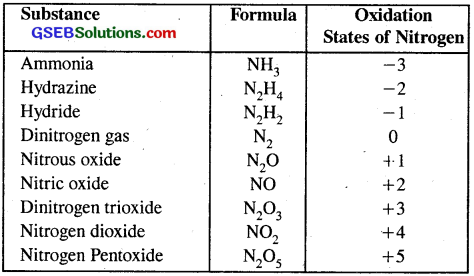Question 8.
While sulphur dioxide & hydrogen peroxide can act as oxidizing as well as reducing agent both in their reactions, ozone, and nitric acid acts only as oxidants. Why?
In both SO2 & H2O2, S & O can increase as well as decrease this oxidation number therefore they can act both as reducing and oxidizing agent. Ozone can only decrease its oxidation state to -1 or -2. so it acts only oxidant.

Oxidation state exhibited by N is +5. In HNO3 nitrogen is present in +5 oxidation state. It can only decrease and not increase. Hence HNO3 can acts as an oxidising agent.Question 9.
Consider the reaction :
(a) 6CO2 (g) + 6H2O (l) → C6H12O6(aq) + 6O2 (g)
(b) O3 (g) + H2O2 (l) → H20(l) + 2O2 (g)
Why it is more appropriate to write these reactions as :
(a) 6CO2 (g) + 12H2O (l) → C6H12O (aq) + 6H2O (l) + 6O2(g)
(b) O3 (g) + H2O2 (l) → H2O(l) + O2 (g) + O2 (g)
Also suggest a technique to investigate the path of the above
(a) & (b) reactions.
(a) It is more appropriate to write down the reaction.
6CO2 (g) + 12H2O (l) → C6H12O6(S) + 6H2O (l) + 6O2(g)
atmospheric CO2, is reduced to C6H12O6 and there are 6 carbon atom reduced. Similarly out of 12 H2O molecules oxidation number of 6O2 atom from 6H2O increase from -2 to 0 in 6O2 molecules whereas in remaining 6H2O molecules its oxidation number remain the same.

(b) It is more appropriate to write down the reaction.
O3 (g) + H2O2 (l) → H2O(l) + 2O2 (g)
because here O3 is reduced to O2 by the reducing action of H2O2.

Question 10.
The compound AgF2 is unstable compound. However if formed, the compound acts as a here strong oxidizing agent. Why?
AgF2 → Ag + F2
Due to the release of F2(g), AgF2 will acts as a very powerful oxidizing agent.Question 11.
Whenever a reaction between an oxidising agent and a reducing agent is carried out, a compound of lower oxidation state is formed if the reducing agent is in excess and a compound of higher oxidation state is formed if the oxidising agent is in excess. Justify this statement giving three illustrations.
Whenever a reaction between an oxidising agent and reducing agent is carried out a compound of lower oxidation state is formed if the reducing agent is in excess and a compound of higher oxidation state is formed if the oxidising agent is in excess. It is clear from the following illustration.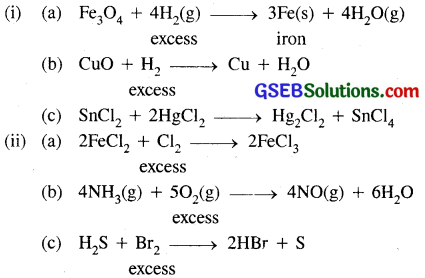Question 12.
How do you count for me following observations?
(a) Though alkaline potassium permanganate and acidic potassium permanganate both are used as oxidants, yet in the manufacture of benzoic acid from toluene we use alcoholic potassium permanganate as an oxidant. Why ? Write a balanced redox equation for the reaction.

(b) When concentrated sulphuric acid is added to an inorganic mixture containing chloride, we get colourless pungent smelling gas HCl, but if the mixture contains bromide then we get red vapour of bromine. Why?
(a) Toluene, being a non-polar liquid will not dissolve in aqueous, alkaline or acidic KMnO4. Therefore alcoholic KMnO4 is preferred in the manufacture of benzoic acid from toluene.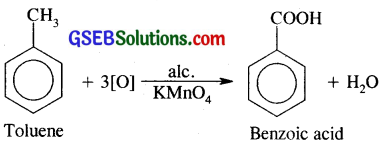(b) When cone. H2SO4 is added to an inorganic mixture containing chloride, it gives colourless pungent smelling HCl gas.
MCI + H2SO4 → MSO4 + HCl
But when cone. H2SO4 is added to inorganic mixture containing bromide we get red vapours of bromine.
MBr + H2SO4 → MHSO4 + HBr
2HBr + O → H2O + Br2

Question 13.
Identify the substance oxidised reduced, oxidising agent an reducing agent for each of the following reactions :
(a) 2AgBr (s) + C6H6O2(aq) → 2Ag(s) + 2HBr (aq) + C6H4O2(aq)

(b) HCHO(l) + 2[Ag(NH3)2]+(aq) + 3OH (aq) → 2Ag(s) + HCOO (aq) + 4NH3(aq) + 2H2O(l)

(c) HCHO (l) + 2Cu2+(aq) + 5 OH (aq) → Cu2O(s) + HCOO(aq) + 3H2O(l)

(d) N2H4(l) + 2H2O2(l) → N2 (g) + 4H2O(l)

(e) Pb(s) + PbO2(s) + 2H2SO4(aq) → 2PbSO4(s) + 2H2O(l)
(a) 2AgBr (s) + C6H6O2(aq) → 2Ag(s) + 2HBr (aq) + C6H4O2 (aq)
AgBr is reduced to Ag
C6H6O2 is oxidised to C6H4O2
AgBr is an oxidizing agent
C6H6O2 is a reducing agent.

(b) HCHO(l) + 2[Ag(NH3)2]+ + 3OH → 2Ag+ + HCOO + 4NH3 + 2H2O (l)
Here HCHO is oxidised to HCOO
[Ag(NH3)2]+ reduced to Ag+
Ag (NH3)2 is an oxidising agent.

(c) HCHO + 2Cu2+ + 5OH → Cu2O + HCOO + 3H2O
HCHO is oxidised to HCOO
Cu2+ is reduced to Cu(l) state.

(d) N2H4 + 2H2O2 → N2 + 4H2O
N2 H4 is reduced to H2O
H2O2 is an oxidising agent.
N2H4 is a reducing agent.

(e) Pb + PbO2 + 2H2SO4 → 2PbSO4 + 2H2O
Pb has been oxidised to PbSO4
PbO2 is reduced to PbSO4
PbO2 is an oxidising agent.
Pb is a reducing agent.Question 14.
Consider the reactions :
2 S2O2-3(aq) + I2(s) → S4O2-6 (aq) + 2I (aq) S2O2-3 (aq) + 2Br2(l) + 5 H2O(l) → 2SO2-4 (aq) + 4Br (aq) + 10H+ (aq)
Why does the same reductant, thiosulphate react differently with iodine and bromine?
In the reactions 2S2 O2-3 (aq) + I2(s) → S4O2-6 + 2I S2 O2-3 (aq) + 2Br2(l) + 5H20(l) → 2SO2-4 + 4Br + 10H+
The reductant i.e. S2O2-3 (aq)
happens to be same but among the halogens Br2 happens to a stronger oxidising agent as compared to I2 which is a much weaker oxidising agent.
The oxidation state of S in S O2-4 is higher than in S4O2-6.

Question 15.
Justify giving reactions that among halogens, fluorine is the best oxidant and among hydrohalic compounds, hydroiodic acid is the best reductant.
Among the halogens best oxidant is F2 and oxidizing character decrease in the order F2 > Cl2 > Br2 > I2
∵ F2 + 2e → 2F(aq) . E0red + 2.87
is maximum among the halogens so much so that F2 can displace Cl > Br > I from their salt solution to Cl2, Br2, I2 respectively.
F2 + 2NaCl(aq) → 2NaF(aq) + Cl2
F2 + 2NaBr(aq) → 2NaF + Br2
F2 + 2NaI(aq) → 2NaF + I2
Cl2 + 2NaF → No reaction.
i. e. F2 can be reduced most readily.
Hence, F2 is the strongest oxidizing agent.
But among the hydrohalic acid HI is the strongest reducing agent.
The reducing power of halogen acids depends upon the ease with which they decompose to give H2 & X2 . This in turn depends upon the bond dissociation energy. Since the bond dissociation energy of the halogen acid increase in the order.
HI < HBr < HCI < HF
∴ reducing power of these acids increase in the reverse direction.
HF < HCI < HBr < HI
2HI ⇌ H2 + I2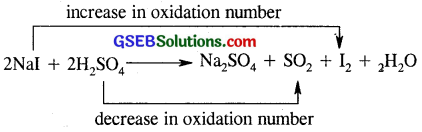Question 16.
Why does the following reaction occur?
XeO4-6 (aq) + 2F (aq) + 6H+ (aq) → XeO3(g) + F2 (g) + 3H2O(l)
What conclusion about the compound Na4XeO6 (of which Xe O4-6 is a part) can be drawn from the reaction.
XeO4-6 (aq) + 2F (aq) + 6H+ (aq) → XeO3(g) + F2 (g) + 3H2O(l)
It is a redox reaction in which Xenon from XeO4-6 is reduce to XeO3.
F is oxidised to F2.
Sodium xenate (Na6 XeO6) is an ionic compound in which oxidation number of sodium does not undergo a change and it is Xenon whose oxidation number change from +8 to +6. It is a powerful oxidising agent which oxidises even F to F2.Question 17.
Consider the reactions;
(a) H3PO2(aq) + 4 AgNO3(aq) + 2 H2O(l) → H3PO4(aq) + 4Ag(s) + 4HNO3(aq)

(b) H3PO2(aq) + 2CuSO4(aq) + 2 H2O(l) → H3PO4(aq) + 2Cu(s) + H2SO4(aq)

(c) C6H5CHO(l) + 2[Ag (NH3)2]+ (aq) + 3OH (aq)
→ C6H5COO (aq) + 2Ag(s) + 4NH3 (aq) + 2 H2O(l)

(d) C6H5CHO(l) + 2Cu2+(aq) + 5OH(aq) → No change observed.
What inference do you draw about the behaviour of Ag+ and Cu2+ from these reactions?
(a) H3PO2(aq) + 4AgNO3(aq) + 2H2O(l) → H3PO4(aq) + 4Ag(s) + 4HNO3(aq)
In this reaction, Ag+ ions have been reduced to Ag. It is oxidizing.
H3PO2 to H3PO4.

(b) H3PO2(aq) + 2CuSO4 + 2H2O(l) → H3PO4(aq) + 2Cu(s) + H2SO4
In this reaction Cu++ ions are reduced to Cu(s)
Thus, it acts as an oxidising agent and oxidises H3PO2 to H3PO4

(c) C6H5CHO(l) + 2[Ag(NH3)2]+(aq) + 30H (aq) → C6H5COO + 2Ag + 4NH3 + 2H2O
In this reaction Ag+ present in the complex [Ag(NH3)2]+ is reduced to Ag(s). It oxidizes C6H5CHO to C6H5COO

(d) C6H5CHO(l) + 2Cu+ (aq) + 5OH (aq) → No change observed.
Here Cu2+ ions are not in a position to oxidise C6H5CHO.

Question 18.
Balance the following redox reactions by ion – electron method :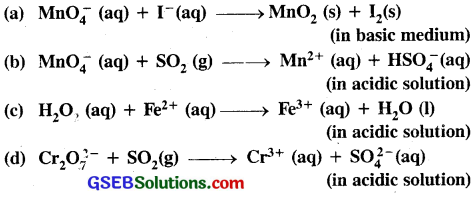(a) MnO4(aq) + I (aq) → MnO2(s) + I2(s) in basic medium.
oxidation half reaction is
(i) I(aq) → I2(s)
(ii) 2I (aq) → I2(s)
(iii) 2I (aq) → I2(s) + 2e … (i)

Reduction half reaction is :
MnO4 → MnO2(s)
(i) MnO4 → MnO2 + 2H2O (To balance O atom).

(ii) MnO4 + 4H2O → MnO2(s) + 2H2O + 4OH (To balance H atom)

(iii) MnO (aq) + 2H2O → MnO2 + 4OH (aq) (Remove duplication)

(iv) MnO4 (aq) + 2H2O + 3e → MnO2 + 40H (aq) … (2)
To equate number of electrons in both half reactions multiply eq. (i) by (3) & equation no. (2) by 2 and add the two half reaction to cancel out electrons.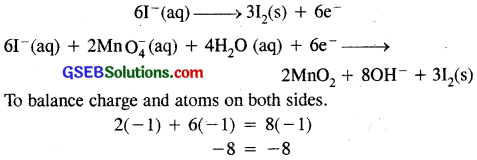(b) MnO4 + SO2(aq) → Mn2+ + HSO4 (aq) (in acidic solution)

oxidation half reaction is :
(i) SO2(g) → HS O4 (aq)
There has occurred an increase in oxidation number
from +4 in SO2 to 6 in HS O4.

(ii)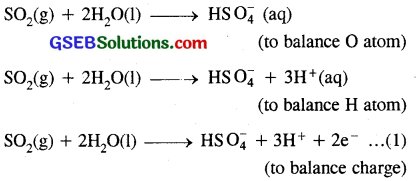Reduction half – reaction is:
(i) MnO4(aq) → Mn2+ (aq)
There has occurred a decrease in oxidation number of Mn from +7 to +2.

(ii)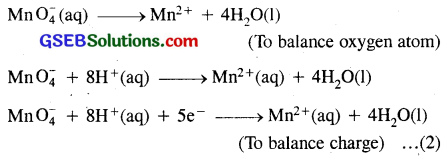Multiply equation (1) by (5) and equation number (2) by 2 to equalise to the number of electrons and add the two half reaction cancelling electrons.
5SO2(g) + 10H2O(l) → 5HS O4 + 15H+ + 10e
2MnO4(aq) + 16H+ (aq) + 10e → -2Mn2+ + 8H2O
2MnO4(aq) + 5SO2(g) + 2H20 + H+ → 2Mn2+ + 5HSO4
To balance atoms and charges
2(-1) + 1 = 2(+ 2) + 5( – 1)
– 1 = – 1

(c) H2O2 (aq) + Fe2+ (aq) → Fe3++(aq) + H2O(l) (In acidic medium)
(i) The oxidation half reaction is
Fe2+ (aq) → Fe3+ (aq)

(ii) Fe2+ (aq) → Fe3+(aq) + e … (1)
The reduction half reaction is
H2O2 (aq) → H20(l)
(Oxidation number of 0 decrease from – 1 in H2O2 to -2 in H2O) (To balance oxygen atoms)

(iii) H2O2(aq) + 2H+(aq) → 2H2O(l) (To balance H atoms in acidic medium)

(iv) H2O2(aq) + 2H+ + 2e → 2H2O(l) … (2) (To balance charge)

(v) Multiply eq. number (1) by 2 to balance the number of electrons in both the half reaction and add them.
2Fe2+ (aq) + H2O2 + 2H+ (aq) → 2Fe3+(aq) + 2H2O(l)
To balance atoms & charge
2(+2) + 2(+1) = 2(+ 3)
+4+2 = +6 + 6 = +6

(d) Cr2O2-7(aq) + SO2(aq) → Cr3+(aq) + SO2-4(aq) (in acidic solution)

Divides the equation in two parts.

Oxidation half reaction –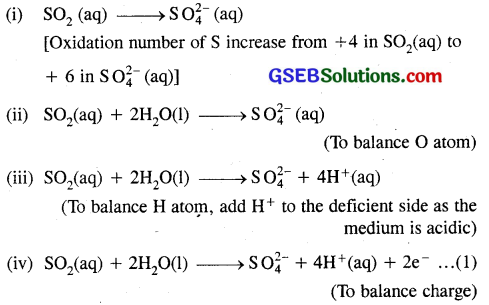Reduction half reaction –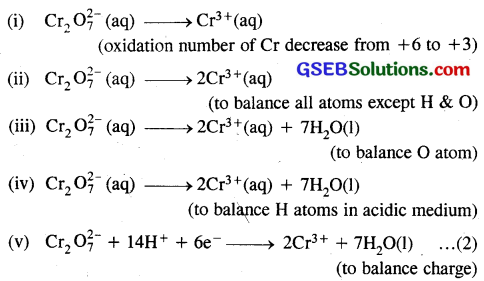(vi) Multiply equation number (1) by 3 to balance electrons in both half reactions & add them to cancel electrons.
Cr2 O2-7(aq) + 14H3+ (aq) + 6e → 2Cr3+ + 7H2O(l)
3SO2(aq) + 6H2O (l) → 3SO2-4 (aq) + 12H+(aq) + 6e
Cr2 O2-7 (aq) + 3SO2(aq) + 2H+(aq) → 2Cr3+ + 3SO2-4 + H2O(l)
– 2 + 2 = + 6 – 6
0 = 0Question 19.
Balance the following equations in basic medium by ion-electron method and oxidation number methods and identify the oxidising agent and the reducing agent.
(a) P4(s) + OH (aq) → PH3(g) + HPO2 (aq)
(b) N2H4(l) + ClO3 (aq) → NO(g) + Cl (g)
(c) Cl2O7 (g) + H2O2(aq) → ClO2 (aq) + O2(g) + H+
Balance the equation by Ion electron method.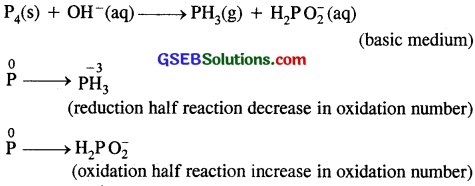oxidation half reaction
P → H2PO2
P + 2OH → H2PO2
P + 2OH → H2PO2 + e … (1)
Reduction half reaction
P → PH3
P + 3H2O → PH3 + 3OH
P + 3H2O + 3e → PH3 + 3OH … (2)
multiply equation (1) by 3 to equalise the number of electrons in both half reaction.
3P + 6OH → 3H2PO2 + 3e
P + 3H2O + 3e → PH3 + 3OH
4P + 3H2O + 3OH → 3H2PO2 + PH3(g)
or P4 + 3H2O(l) + 3OH (aq) → 3H2PO2 + PH3(g)
Balance the atoms and charge on both sides
3(- 1) = +3( -1)
-3 = -3
It is a disproportionation reaction.
Here phosphorus undergoes oxidation as well as reduction. Therefore P is both an oxidant & as a reductant.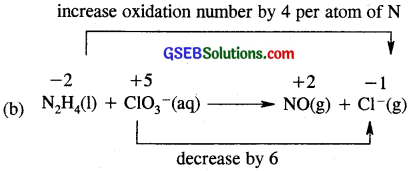N ↑ 8 x 3
Cl ↑ 6 x 4
3N2H4(l) + 4ClO3 (aq) → 6NO + 4Cl
Now balance H and O atom
3N2H4(1) + 4ClO3 (aq) → 6NO(g) + 4Cl + 6H2O
4(- 1) = 4(- 1)
– 4 = – 4
Here Cl O3 ions is an oxidant and N2H4 is a reductant.

(c)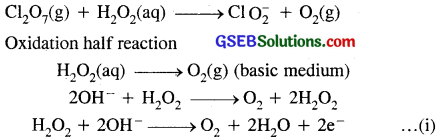Reduction half reaction
Cl2O7(g) → ClO2
Cl2O7(g) → 2ClO2
Cl2O7(g) → 2ClO2 + 3H2O
Cl2O7(g) + 6H2O(l) → 2ClO2 + 3H2O + 6OH
Cl2O7(g) + 3H2O(l) → 2ClO2 + 6OH
Cl2O7(g) + 3H20 + 8e → 2ClO7 + 6OH
To equalise electrons in both half reactions multiply equation number (1) by 4. Add both the half reactions to cancel out electrons.
4H2O2(aq) + 80H(aq) → 4O2 + 8H2O(l) + 8e
Cl2O7 + 3H2O(l) + 8e
Cl2O7(g) + 4H2O (aq) + 2OH (aq) → 2ClO2 (aq) + 5H2O + 4O2
To balance atoms and charges
2(- 1) = 2( -1)
– 2 = – 2
In this reaction, Cl2O7 is an oxidising agent and H2O2 acts as a reducing agent.

Question 20.
What sorts of informations can you draw from the following reaction?
(CN)2(g) + 2OH(aq) → CN(aq) + CNO(aq) + H2O(l)
(CN)2 (g) + 2OH(aq) → CN(aq) + CNO + H2O(l)
(i) In the above reaction (CN)2 is called a pseudohalogen while CN, CNO ions are called Pseudohalides ions.

(ii) Ions which consist of two or more electronegative atoms of which atleast one is nitrogen and have properties similar to those of halides ions are called pseudohalides ions.

(iii) Just as halide ions (Cl) have corresponding diatomic molecules (Cl2) pseudohalides ions (CN, CNO) have also corresponding dimeric molecules (CN)2, (CNO)2. These are called pseudohalogens and show properties similar to those of halogens. (CN)2 is cyanogen and similar to Cl2.

(iv) They undergoes reactions similar to halogens as Cl2(g) disproportionate to give Cl ions and ClO ions in basic medium. So does (CN) does above.

Question 21.
The Mn3+ ion is unstable in solution and undergoes disproportionation to give Mn2+, MnO2, and H+ ion. Write a balanced ionic equation for the reaction.
The skeletal equation is :
Mn3+ → Mn2+ + MnO2 + H+ (aq)
Oxidation half reaction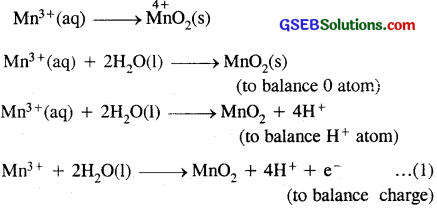Reduction half reaction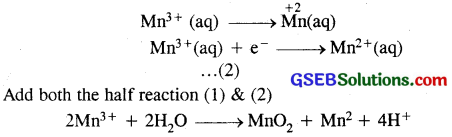It is the net balanced ionic equation
2(+ 3) = +2 + 4(+1)
+6 = +6Question 22.
Consider the elements :
Cs, Ne, I and F
(a) Identify the element that exhibits only negative oxidation state.
(b) Identify the element that exhibits only positive oxidation state.
(c) Identify the element that exhibits both positive and negative oxidation states.
(d) Identify the element which exhibits neither the negative nor does the positive oxidation state.
(a) F exhibit only -ve oxidation state (F).
(b) Cs exhibit only +ve oxidation state (CS+).
(c) I exhibit both -ve & +ve oxidation state I & I+
(d) Ne neither shows +ve oxidation state nor does it shows -ve oxidation state.

Question 23.
Chlorine is used to purify drinking water. Excess of chlorine is harmful. The excess of chlorine is removed by treating with sulphur dioxide. Present a balanced equation for this redox change taking place in water.
Cl2(g) + SO2(aq) + 2H2O(l) → 2HCl(aq) + H2SO4(aq)
Here Cl2(g) undergoes reduction to form HCl whereas SO2 undergoes oxidation to form H2SO4.

Question 24.
Refer to the periodic table given in your book and now answer the following questions:
(a) Select the possible non metals that can show disproportionation reaction.
(b) Select three metals that can show disproportionation, reaction.
(a) Oxygen, chlorine, bromine, phosphorus etc.
(b) Copper, manganese.

Question 25.
In Ostwald’s process for the manufacture of nitric acid, the first step involves the oxidation of ammonia gas by oxygen gas to give nitric oxide gas and steam.
What is the maximum weight of nitric oxide that can be obtained starting only with 10.00 g. of ammonia and 20.00 g of oxygen ?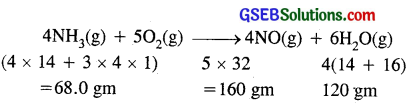not of NH3 given = 10.00 gm
not of O2 given = 20 gm.
Let us arbitrarily choose NH3 which will react with 20 gm of O2.
Mass of NH3 required = $$\frac { 60 }{ 160 }$$ x 20 = 8.5 gm
It means 20 gm of O2 will require 8.5 gm of NH3.
∴ Oxygen must be the limiting reactant and NH3 is present in excess i.e. 10 gm.
Now if the entire mass of O2 react how much NO is formed.
Mass of NO produce from 20 gm of O2
$$\frac { 120 }{ 160 }$$ x 20 = 15 gm of NO.
Maximum wt. of NO produced = 15 gm.

Question 26.
Using the standard electrode potentials given in the Table 8.1, predict if the reaction between the following is feasible:
(a) Fe3+ (aq) and I (aq)
(b) Ag3+ (aq) and Cu(s)
(c) Fe3+ (aq) and Cu(s)
(d) Ag(s) and Fe3+ (aq)
(e) Br (aq) and Fe2+ (aq).
(a) Fe3+ (aq) and I (aq)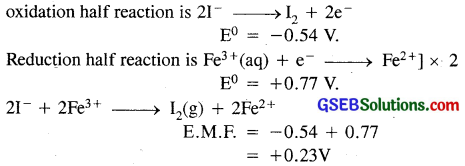since the EMF is positive, the reaction is feasible.

(b) Here Cu(s) loses electrons and Ag+(aq) gains electrons. Thus,
oxidation half cell reaction is
Cu(s) → Cu2+ + 2e] E° = – 0.34V
Reduction Ag+ + e → Ag(s) x 2 E° = – 0.80 V
overall reaction is :
Cu(s) + 2Ag+ → Cu2+ (aq) + 2Ag(s)
cell = – 0.46V
Since E°cell is positive the reaction is feasible.

(c) Oxidation Cu(s) → Cu2+(aq) + 2e, E° = – 0.34V
Reduction Fe34 + e → Fe2+ E° = +0.77V
Probable cell
Cu(s), Cu2+ : : Fe3+, Fe2+
cell = E°red(R.H.S) – E°oxx L.H.S.
= [+0.77 – 0.34] V = 0.43V.
since E°cell is positive, reaction is feasible.

(d) Ag (s) and Fe3+ (aq)
Oxidation Ag(s) → Ag+ + e E° = – 0.80V
Reduction Fe3+ + e → Fe2+, E° = + 0.77V
cell = – 0.03V
since E°cell is negative, reaction is not feasible.

(e) Br2 and Fe2+
Here Fe2+ lose electrons and Br2 gains them.
Oxidation Fe2+ → Fe3+ + e] x 2 [E° = 0.77V
Reduction Br2 + 2e → – 2Br] (E° = + 1.08V)
cell = +0.31V
Reaction is 2Fe2+ + Br2 → 2Fe3+ + 2Br
since E°cell is positive i.e. reaction is feasible.Question 27.
Predict the products of electrolysis in each of the following:
(i) An aqueous solution of AgNO3 with silver electrodes
(ii) An aqueous solution of AgNO3 with platinum electrodes
(iii) A dilute solution of H2SO4 with platinum electrodes
(iv) An aqueous solution of CuCl2 with platinum electrodes.
(i) AgNO3 ⇌ Ag+ + NO3
At cathode Ag+ + e → Ag silver is deposited
At anode = NO3 ions move, Dissolve, equivalent amount of Ag concentration AgNO3 remains unchanged.

(ii) At cathode : Ag will be liberated.
At anode : O2 gas will be liberated.

(iii) At anode : O2 gas will be liberated
At cathode : H2 gas will be liberated.

(iv) At cathode : Cu will be liberated.
At anode : Cl2 gas will be liberated.

Question 28.
Arrange the following metals in the order in which hey displace each other from the solution of their salts.
Al, Cu, Fe, Mg and Zn.
Any metal will displace another metal from its salt solution is dependent on the values of their reduction potential values.
The order of displacement is thus :
Mg = – 2.37
Al = – 1.66
Zn = – 0.76
Fe = + 0.44
Cu = + 0.34
Thus, the decreasing order is :
Mg < Al < Zn < Fe < Cu

Question 29.
Given the standard electrode potentials,
(i) K+/K = – 2.93V
(ii) Mg2+/Mg = – 2.37V
(iii) Ag+/Ag = 0.80V
(iv) Hg2+ /Hg = 0.79V
(v) Mg2+/Mg = – 2.37V. Cr3+/Cr = – 0.74V
arrange these metals in their increasing order of reducing power.
(i) K+/K = – 2.93V
(ii) Mg2+/Mg = – 2.37V
(iii) Cr3+/Cr = – 0.74V
(iv) Hg2+/Hg = 0.79V
(v) Ag+/Ag = 0.80V
The Lower the reduction potential more the tendency to get oxidised and thus more will be reducing power.
Hence increasing order of reducing power is.:
Ag > Hg > Cr > Mg > KQuestion 30.
Depict the galvanic cell in which the reaction
Zn(s) + 2Ag+(aq) → Zn+(aq) +2Ag(s) takes place,
Further show:
(i) which of the electrode is negatively charged,
(ii) the carriers of the current in the cell, and
(iii) individual reaction at each electrode.